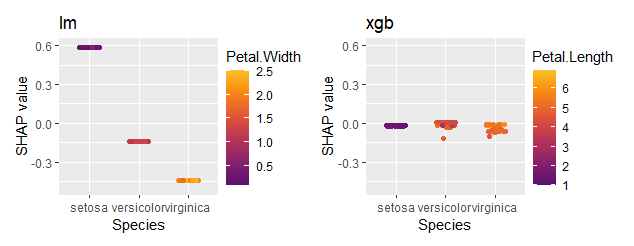# Multiple ‘shapviz’ objects

## Overview

Sometimes, you will find it necessary to work with several “shapviz” objects at the same time:

• To visualize SHAP values of a multiclass or multi-output model.
• To compare SHAP plots of different models.
• To compare SHAP plots between subgroups.

To simplify the workflow, {shapviz} introduces the “mshapviz” object (“m” like “multi”). You can create it in different ways:

• Use shapviz() on multiclass XGBoost or LightGBM models.
• Use shapviz() on “kernelshap” objects created from multiclass/multioutput models.
• Use c(Mod_1 = s1, Mod_2 = s2, ...) on “shapviz” objects s1, s2, …
• Or mshapviz(list(Mod_1 = s1, Mod_2 = s2, ...))

The sv_*() functions use the {patchwork} package to glue the individual plots together.

## Example: Multiclass model

### Fit classification model

library(xgboost)
library(ggplot2)
library(shapviz)
library(patchwork)

params <- list(objective = "multi:softprob", num_class = 3)
X_pred <- data.matrix(iris[, -5])
dtrain <- xgb.DMatrix(X_pred, label = as.integer(iris[, 5]) - 1)
fit <- xgb.train(params = params, data = dtrain, nrounds = 50)

### Create “mshapviz” object (logit scale)

Note that TreeSHAP produces SHAP values on link scale, i.e., on logit scale.

x <- shapviz(fit, X_pred = X_pred, X = iris)
x
#> 'mshapviz' object representing 3 'shapviz' objects:
#>    'Class_1': 150 x 4 SHAP matrix
#>    'Class_2': 150 x 4 SHAP matrix
#>    'Class_3': 150 x 4 SHAP matrix

# Contains "shapviz" objects for all classes
all.equal(x[], shapviz(fit, X_pred = X_pred, X = iris, which_class = 3))
#>  TRUE

# Better names
names(x) <- levels(iris$Species) x #> 'mshapviz' object representing 3 'shapviz' objects: #> 'setosa': 150 x 4 SHAP matrix #> 'versicolor': 150 x 4 SHAP matrix #> 'virginica': 150 x 4 SHAP matrix ### Force plot sv_force(x, row_id = 101)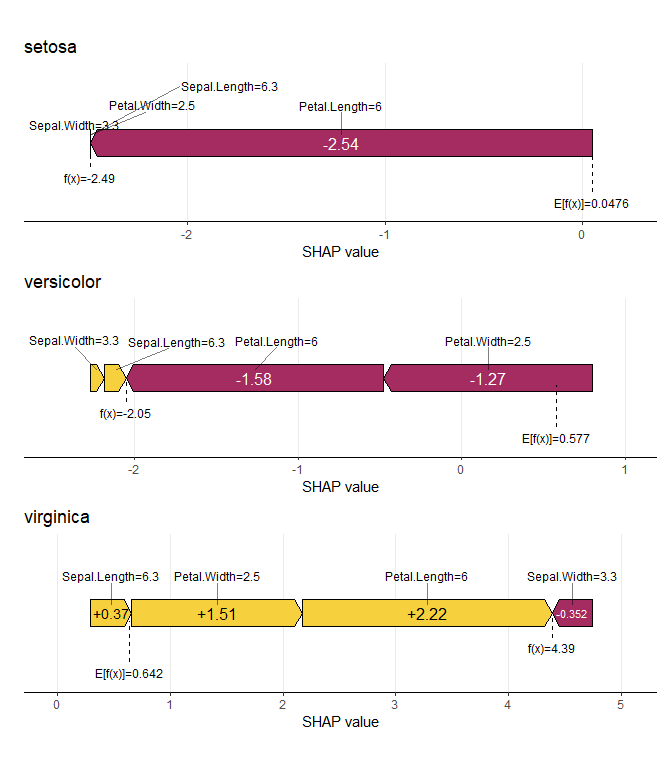### Summary plot sv_importance(x, kind = "bee") + plot_layout(ncol = 1)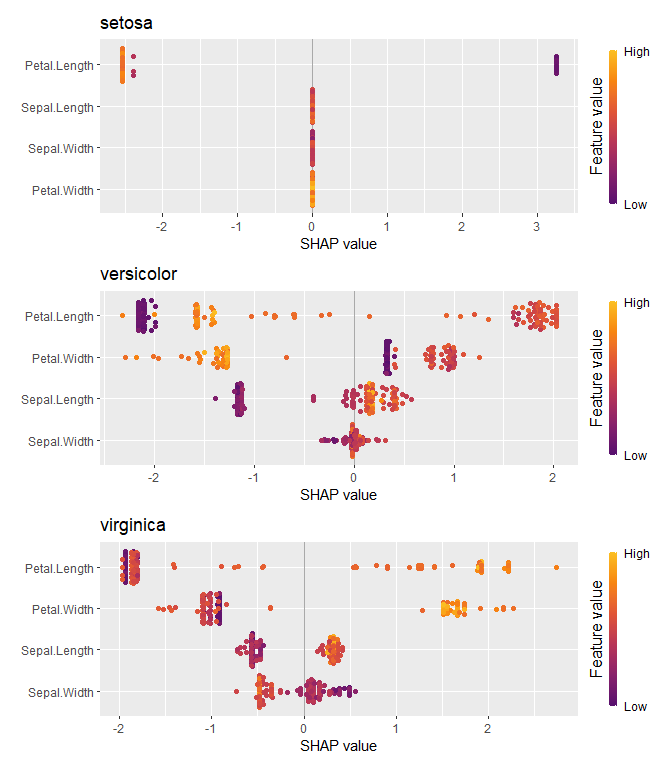### Dependence plot Here, we will use identical coordinate systems. names(x) <- levels(iris$Species)

sv_dependence(x, v = "Petal.Length") +
plot_layout(ncol = 1) &
xlim(1, 7) &
ylim(-3, 4)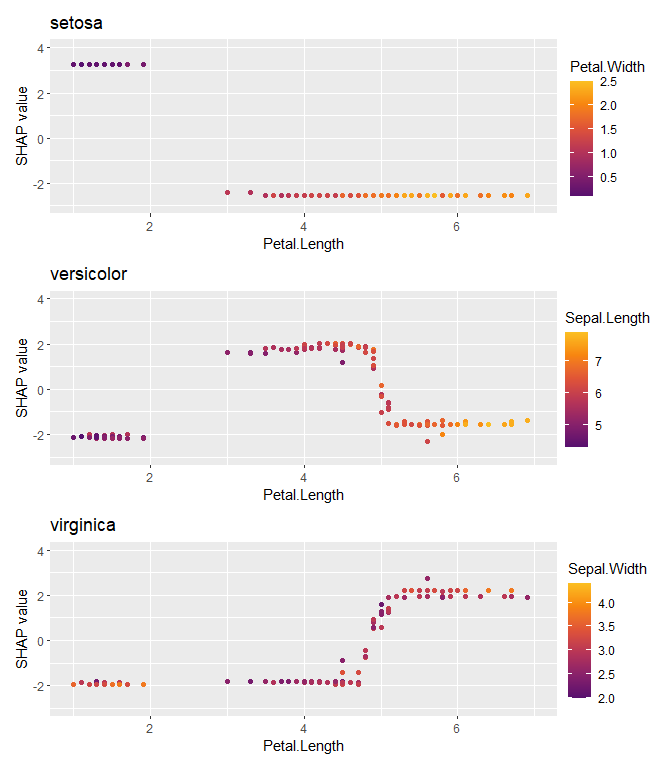### Similar for LightGBM (only code)

library(lightgbm)

# Model
params <- list(objective = "multiclass", num_class = 3)
X_pred <- data.matrix(iris[, -5])
dtrain <- lgb.Dataset(X_pred, label = as.integer(iris[, 5]) - 1)
fit <- lgb.train(params = params, data = dtrain, nrounds = 50)

x <- shapviz(fit, X_pred = X_pred, X = iris)

sv_importance(x, show_numbers = TRUE) +
plot_layout(ncol = 1) &
xlim(0, 2.25)

### And for random forest and kernelshap

Since Kernel SHAP is model agnostic, we can get SHAP values on probability scale.

library(ranger)
library(kernelshap)

# Model
fit <- ranger(Species ~ ., data = iris, num.trees = 100, probability = TRUE)

# "mshapviz" object
x <- kernelshap(fit, X = iris[-5], bg_X = iris)

### Dependence plot

sv_dependence(x_subgroups, v = "Petal.Length") +
plot_layout(ncol = 1) &
xlim(1, 7) &
ylim(-1.4, 2.2)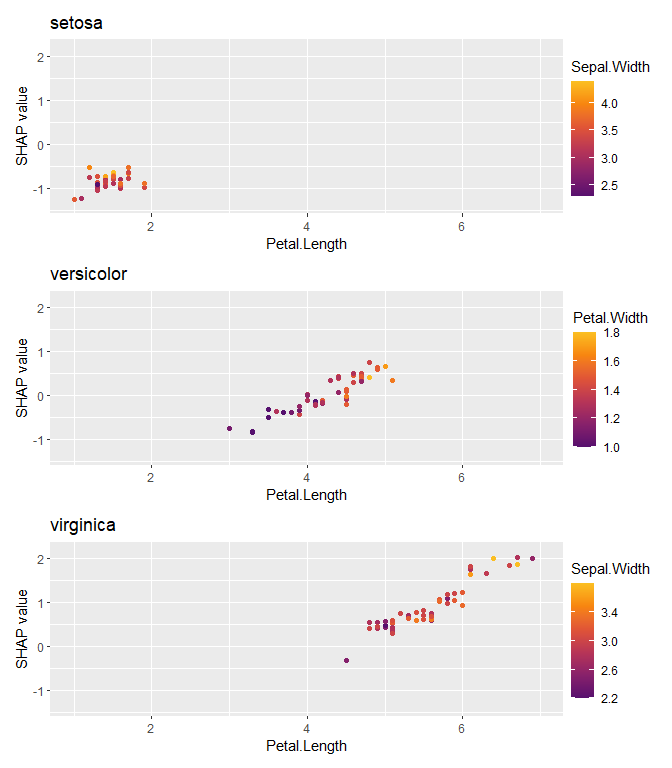## Example: Different models

In the last example, we used a regression model fitted via XGBoost. How does it compare with a linear regression?

### Fit linear regression and use {kernelshap} to get SHAP values

library(kernelshap)

fit_lm <- lm(Sepal.Length ~ ., data = iris)
shap_lm <- shapviz(kernelshap(fit_lm, iris[-1], bg_X = iris))

### Combine “shapviz” objects

mshap <- c(lm = shap_lm, xgb = shap_xgb)
mshap

#> 'mshapviz' object representing 2 'shapviz' objects:
#>   'lm': 150 x 4 SHAP matrix
#>   'xgb': 150 x 4 SHAP matrix

### SHAP importance plot

sv_importance(mshap)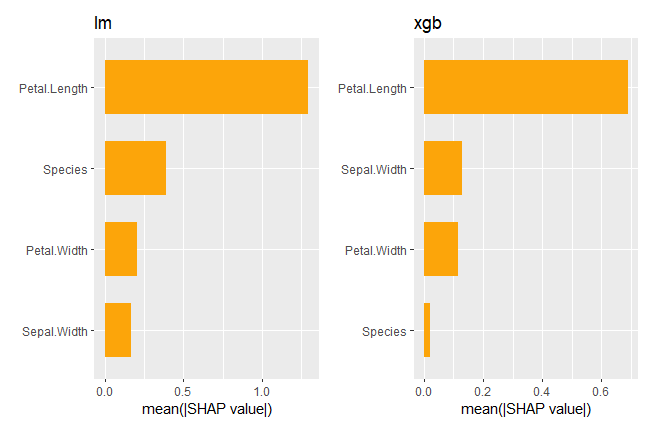### SHAP dependence plot

sv_dependence(mshap, v = "Species") &
ylim(-0.5, 0.6)